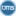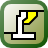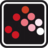# Wolfram Mathematica

12.0.0

Mathematica is a technical computing software package. It has nearly 5000 built-in functions covering various areas of technical computing; all of them are carefully integrated so that they work perfectly together. It uses the Wolfram Language for writing code.

3.7
Rated by
94 users
Shareware \$172
OS:
Windows 7/8.1/10
Date:
File size:
71.94 MB
Category rank:
8
All software rank:
844

Get Wolfram Mathematica v12.0.0; you will need 71.94 MB for the installation on the hard drive; Mathematica and Wolfram Mathematica (M-WIN-L 7.0.0 1148351) are its known aliases. Wolfram Research, Inc. distributes this program on a trial basis providing the full version for \$172. The software is a part of the category Education with the Science subcategory and supports .m, .ma, .nb, .nbp formats. The software versions that users like running on Windows 7/8.1/10 are 12.0 and 11.3. The statistics show that Mathematica.exe and WolframMathematica.exe are among the most popular setup files for this tool. The antivirus system of our website marked the download link as safe to use.Download
Wolfram Mathematica 12.0.0

Thank you for rating the program!

### Useful softwareWolfram Mathematica for Students
Mathematica is ideal for students enrolled in a variety of courses.Mosek Optimization Tools
This app is designed to solve large-scale mathematical optimization problems.